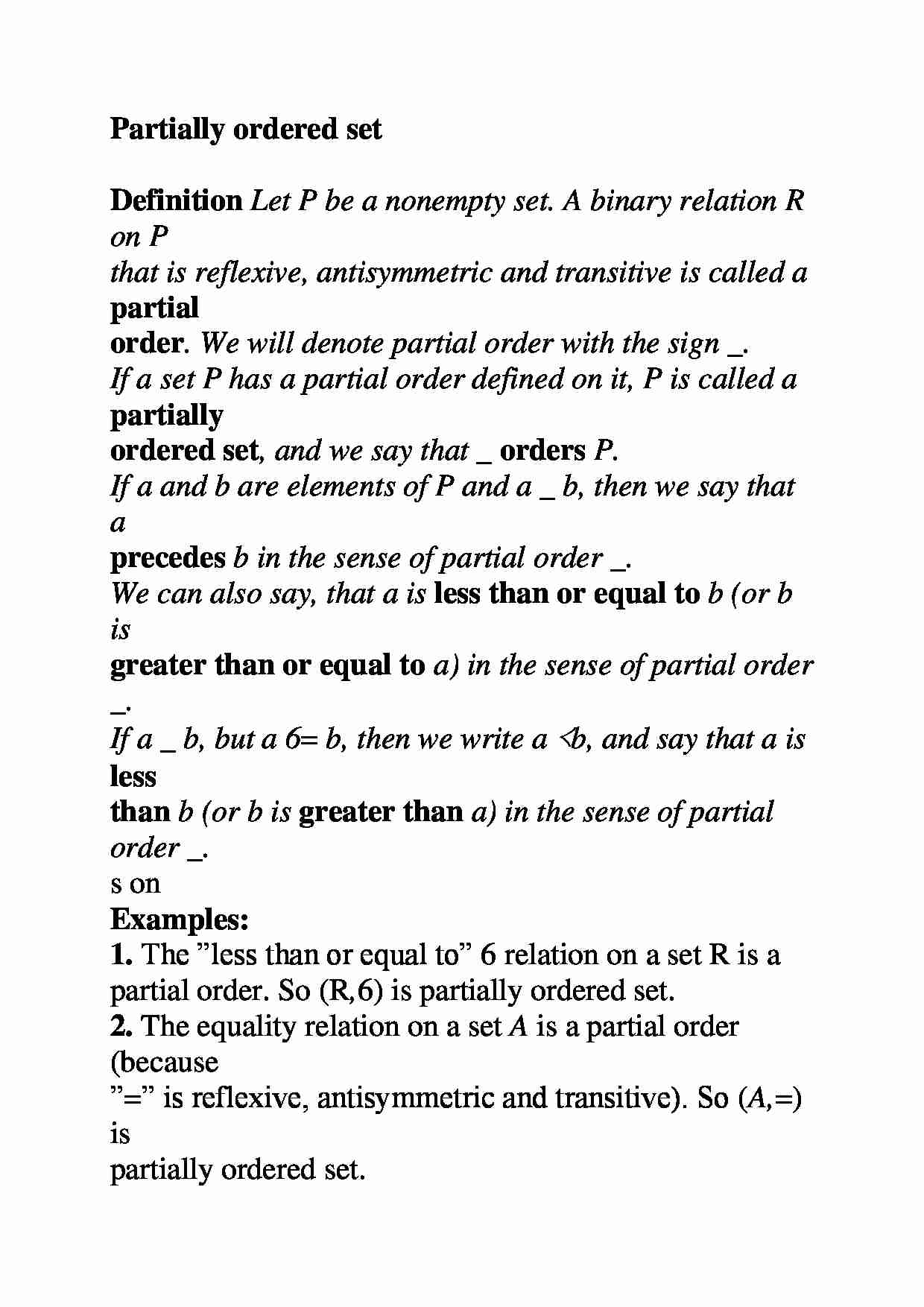# Częściowo uporządkowany zestaw

Nasza ocena:

Wyświetleń: 588
Komentarze: 0

### Pobierz ten dokument za darmo

Podgląd dokumentu## Fragment notatki:

Partially ordered set Definition Let P be a nonempty set. A binary relation R on P that is reflexive, antisymmetric and transitive is called a partial order . We will denote partial order with the sign _ . If a set P has a partial order defined on it, P is called a partially ordered set , and we say that _ orders P . If a and b are elements of P and a _ b , then we say that a precedes b in the sense of partial order _ . We can also say, that a is less than or equal to b (or b is greater than or equal to a ) in the sense of partial order _ . If a _ b , but a 6 = b , then we write a ≺ b , and say that a is less than b (or b is greater than a ) in the sense of partial order _ . s on Examples: 1. The ”less than or equal to” 6 relation on a set R is a partial order. So ( R , 6 ) is partially ordered set. 2. The equality relation on a set A is a partial order (because ” = ” is reflexive, antisymmetric and transitive). So ( A, =) is partially ordered set. 3. Let S be a set. The subset relation on P ( S ) (power set of S ) is a partial order. So ( P ( S ) , ⊂ ) is partially ordered set. 4. Let Z be a set of all integers. The relation ” m divides n ” is a partial order on Z . So ( Z , | ) is partially ordered set. Relations on 5. Let P be an alphabet, and suppose, that P is a partially ordered set, with partial order _ . One can use the relation _ to define the partial order on the set P ⋆ . To compare two words w = a 1 , a 2 , ..., a n and u = b 1 , b 2 , ..., b k we first compare their first letters: - if a 1 ≺ b 1 , then we have w _ u . If a 1 = b 1 , then we compare the second letters in each words; - if a 1 = b 1 and a 2 ≺ b 2 , then we have w _ u . If a 1 = b 1 and a 2 = b 2 , then we compare the third letters in each words, and so on; - if w is a prefix of u , then w _ u ; - if w = u , then w _ u or u _ w ; - ? _ w - empty word ? precedes every other word in order. The partial order _ defined in this way on P ⋆ is called a lexicographic order or dictionary order . 6. Lexicographic order can be used to order the set R 2 of all ordered pairs in the plane - one have to think of an ordered pair ( a, b ) as a word of length 2. So, the lexicographic order is defined as follows: ( a, b ) _ ( c, d ) ⇔ ... zobacz całą notatkę

## Komentarze użytkowników (0)

Zaloguj się, aby dodać komentarz